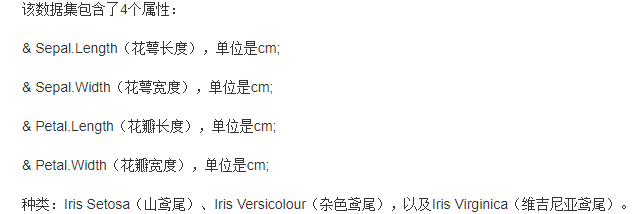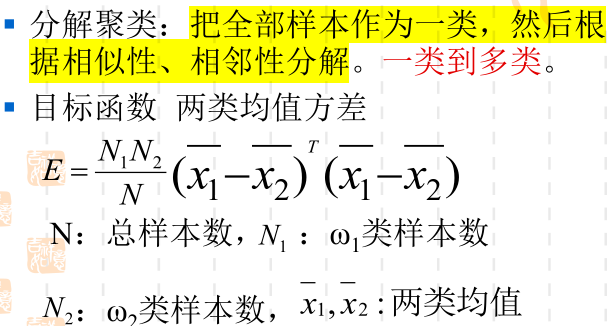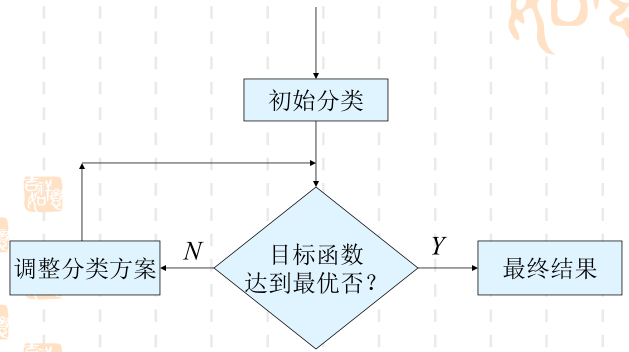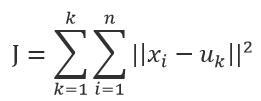• 聚类分析常用数据集

千次下载 热门讨论 2014-03-26 09:21:18
聚类分析常用的人工数据集，包括：UCI:wine、Iris、yeast，还有4k2_far、leuk72_3k等数据集。它们在聚类分析、数据挖掘、机器学习、模式识别领域经常用到。
• 二维多维不同簇数的点集，螺旋分布、月牙分布、环形分布等数据集，共30余种数据挖掘 机器学习
• 基于项目提供的汽车相关数据，通过聚类分析的方法实现汽车产品聚类，以构建汽车产品画像、分析产品定位、完成汽车竞品分析等要求。 2. 项目数据 项目提供的汽车数据包括26个字段共205条数据数据文件为“car_price...
• 全部为txt文档数据，数据挖掘聚类分析技术，算法实验过程中，经常用到的经典UCI、UPSP等真实数据集，常用于算法的实验验证。文档中注有相应的数据量、属性等信息，可直接进行使用。
• 这里面是机器学习里面聚类所需的数据集，分为人工的二维数据集，如月牙形，双螺旋型等，和UCI真实数据集，是我搜集好久才弄出来的，有一些二维数据集是自己生成的，提供给大家做算法实验。聚类数据集
• 聚类分析、机器学习、数据挖掘中常用到的数据集，包括：UCI的数据集wine,yeast,iris等，还有USPS数据集，4k2_far,leuk72_3k数据集等。
• UCI数据集有好多种类，不知道选什么好，貌似Clustering和Classfication类别下的都可以？这两个类别有什么区别啊，感觉都是分类呀？ 不是UCI上的也可以给我推荐一下，比较迷茫，最好是二维的数据。数据集 测试 聚类
• 机器学习中常用的聚类数据集生成方法

千次阅读 多人点赞 2021-05-26 11:51:40
毕业季又到了，论文里涉及到一些机器学习算法，就想用一些数据样本来对算法的性能做一些可视化，苦于自制数据集的困难，所以本篇就介绍一下scikit-learn自带的一些函数库来生成数据。

前言

毕业季又到了，论文里涉及到一些机器学习算法，就想用一些数据样本来对算法的性能做一些可视化，苦于自制数据集的困难，所以本篇就介绍一下scikit-learn自带的一些函数库来生成数据。

1. 造轮子式生成数据样本

很久之前，也就是对scikit-learn不是特别熟悉的时候，那时候找不到合适的数据集，于是就用numpy自己造轮子，过程之艰难就不一一细说了，先看一个自己做的。

1.1 月牙形

import numpy as np

np.random.seed(256)

x1 = np.linspace(-3, 3, 300, dtype=np.float32)[:, np.newaxis]
noise = np.random.normal(0, 0.15, x1.shape).astype(np.float32)
y1 = -np.square(x1) / 3 + 4.5 + noise

x2 = np.linspace(0, 6, 300, dtype=np.float32)[:, np.newaxis]
y2 = np.square(x2 - 3) / 3 + 0.5 + noise

plt.figure()
plt.xticks([])
plt.yticks([])
plt.scatter(x1, y1, s=15)
plt.scatter(x2, y2, s=15)

plt.show()1.2 方形

import numpy as np

np.random.seed(256)

x = np.random.rand(1000, 2)
condition1 = x[:, 1] <= x[:, 0]
condition2 = x[:, 1] <= (1-x[:, 0])
index1 = np.where(condition1 & condition2)
x1 = x[index1]
x = np.delete(x, index1, axis=0)
index2 = x[:, 0] <= 0.5
x2 = x[index2]
x3 = np.delete(x, index2, axis=0)

plt.figure()
plt.xticks([])
plt.yticks([])
plt.scatter(x1[:, 0], x1[:, 1], s=20)
plt.scatter(x2[:, 0], x2[:, 1], s=20)
plt.scatter(x3[:, 0], x3[:, 1], s=20)
plt.show()1.3 螺旋形

n_samples = 1500
np.random.seed(0)

t = 1.5 * np.pi * (1 + 3 * np.random.rand(1, n_samples))
x = t * np.cos(t)
y = t * np.sin(t)

X = np.concatenate((x, y))
X += 0.7 * np.random.randn(2, n_samples)
X = X.T

norm = plt.Normalize(y.min(), y.max())

plt.figure()
plt.xticks([])
plt.yticks([])
# cmap=plt.cm.nipy_spectral
plt.scatter(X[:, 0], X[:, 1], s=20, c=norm(X[:, 0]), cmap='viridis')
plt.show()还可以，是吧，想要什么形状的数据都可以自己造，无非就是拟合一个函数再加上噪声，但就是稍微有一些繁琐，不如现成的库函数来的实在。当然啦，如果没事干，自己去探索不同的方程然后用代码的形式去实现，还是蛮好的。

2. 自定义生成数据样本

使用sklearn.datasets自带的样本生成器来做数据集，具体参数可以参考官方文档

2.1 点簇形

from sklearn.datasets import make_blobs

# x1表示生成的样本数据	y1表示样本的标签
x1, y1 = make_blobs(n_samples=300, n_features=2, centers=3, random_state=170)

plt.figure()
plt.xticks([])
plt.yticks([])
plt.scatter(x1[:, 0], x1[:, 1], c=y1, s=20)
plt.show()一条命令就可以生成数据，通过修改参数即可制作不同的数据集。如果颜色不喜欢，还可以修改一下：

x1, y1 = make_blobs(n_samples=300, n_features=2, centers=3, random_state=170)

plt.figure()
plt.xticks([])
plt.yticks([])
# plt.scatter(x, y, c=None, s=None)
# c 表示点的颜色	s表示点的大小
plt.scatter(x1[y1==0][:, 0], x1[y1==0][:, 1], s=20)
plt.scatter(x1[y1==1][:, 0], x1[y1==1][:, 1], s=20)
plt.scatter(x1[y1==2][:, 0], x1[y1==2][:, 1], s=20)
plt.show()2.2 线簇形

圆簇形状的不喜欢的话还可以把它拉直一下，做一个变换：

x1, y1 = make_blobs(n_samples=300, n_features=2, centers=3, random_state=170)
transformation = [[0.60834549, -0.63667341], [-0.40887718, 0.85253229]]
x1 = np.dot(x1, transformation)

plt.figure()
plt.xticks([])
plt.yticks([])
plt.scatter(x1[y1==0][:, 0], x1[y1==0][:, 1], s=20)
plt.scatter(x1[y1==1][:, 0], x1[y1==1][:, 1], s=20)
plt.scatter(x1[y1==2][:, 0], x1[y1==2][:, 1], s=20)
plt.show()2.3 环形

from sklearn.datasets import make_circles

x1, y1 = make_circles(n_samples=500, noise=0.07, random_state=16, factor=0.6)

plt.figure()
plt.xticks([])
plt.yticks([])
plt.scatter(x1[y1==0][:, 0], x1[y1==0][:, 1], s=20)
plt.scatter(x1[y1==1][:, 0], x1[y1==1][:, 1], s=20)
plt.show()2.4 圆形

调整一下画板的尺寸，还可以变成圆形的形状：

x1, y1 = make_circles(n_samples=500, noise=0.07, random_state=16, factor=0.6)

plt.figure(figsize=(6, 6))
plt.xticks([])
plt.yticks([])
plt.scatter(x1[y1==0][:, 0], x1[y1==0][:, 1], s=20)
plt.scatter(x1[y1==1][:, 0], x1[y1==1][:, 1], s=20)
plt.show()2.5 月牙形

from sklearn.datasets import make_moons

x1, y1 = make_moons(n_samples=300, noise=0.07, random_state=120)

plt.figure()
plt.xticks([])
plt.yticks([])
plt.scatter(x1[y1==0][:, 0], x1[y1==0][:, 1], s=20)
plt.scatter(x1[y1==1][:, 0], x1[y1==1][:, 1], s=20)
plt.show()结束语

sklearn里面还有好多函数来自定制数据，除此之外还可以使用numpy生成，然后通过高级索引进行划分，最好结合着matplotlib中的cmap来做颜色映射，这样可以做出好玩又好看的数据集。

展开全文python 机器学习 可视化 numpy sklearn
• 一些用于聚类和分类问题的数据集

万次阅读 多人点赞 2018-08-13 15:02:57
毕业设计时简单研究了聚类和分类问题，整理了一下用到的数据集，有需要的可以参考一下。。。 聚类数据集信息 序号 数据集 记录数 特征数 类别 简单分布 是否有overlap 来源 1 iris 150 4 3 50...

毕业设计时简单研究了聚类和分类问题，整理了一下用到的数据集，有需要的可以参考一下。。。

聚类数据集信息

序号数据集记录数特征数类别简单分布是否有overlap来源
1iris1504350/50/50NoUCI
2wine17813359/71/48NoUCI
3emotions(music)593726173/166/264/148/168/189YESsourceforge
4yeast241710314混合分布YESsourceforge
5scene24072946427/364/397/433/533/431YESsourceforge
6wdbc569302212/357NoUCI
7breasttissue1069621/15/18/16/14/22NoUCI
8seeds2107370/70/70NoUCI
9glass21496(7)70/76/17/13/9/29NoUCI

分类数据集信息

序号数据集记录数特征数类别简单分布是否有缺失值来源
1appendicitis1067221/85NoKEEL
2balance62543288/49/288NoKEEL,UCI
3banana5300222924/2376NoKEEL
4bands365(539)192230/135YesKEEL,UCI
5bupa34562145/200NoKEEL,UCI
6cleveland297(303)135160/54/35/35/13YesKEEL,UCI
7dermatology358(366)346111/60/71/48/48/20YesKEEL,UCI
8haberman30632225/81NoKEEL,UCI
9hayes-roth1604365/64/31NoKEEL,UCI
10heart270132150/120NoKEEL,UCI
11hepatitis80(155)19213/67YesKEEL,UCI
12ionosphere351342225/126NoKEEL,UCI
13iris1504350/50/50NoKEEL,UCI
14led7digit50071045/37/51/57/52/52/47/57/53/49NoKEEL,UCI
15mammographic830(961)52427/403NoKEEL,UCI
16marketing6876(8993)1391255/529/505/618/527/846/784/1069/743YesKEEL,biolab
17monks243272290/142NoKEEL,UCI
18movement_libras360901524/…/24NoKEEL,UCI
19newthyroid21553150/35/30NoKEEL,UCI
20pageblocks54731054913/329/28/88/115NoKEEL,UCI
21penbased100921610NoKEEL,UCI
22phoneme5404523818/1586NoKEEL,UCL
23pima76882500/268NoKEEL,UCI
24ring74002023664/3736NoKEEL,UTO
25satimage64353671533/703/1358/626/707/0/1508NoKEEL,UCI
26segment2310197330/…/330NoKEEL,UCI
27sonar20860297/111NoKEEL,UCI
28spambase4597(4601)5722788/1813YesKEEL,UCI
29spectfheart26744255/212NoKEEL,UCI
30tae1515349/50/52NoKEEL,UCI
31texture55004011500/…/500NoKEEL,UCL
32thyroid7200213166/368/6666NoKEEL,UCI
33titanic2201321490/711NoKEEL,TOR
34twonorm74002023703/3697NoKEEL,UTO
35vehicle846184212/218/199/217NoKEEL,UCI
36vowel990131190/…/90NoKEEL,UCI
37wdbc569302212/357NoUCI
38wine17813359/71/48NoUCI
39winequality-red1599111110/53/681/638/199/18NoKEEL,UCI
40wisconsin683(699)92444/239NoKEEL,UCI
展开全文聚类 分类 数据集
• 将分解聚类、C均值聚类及其改进、ISODATA、K均值聚类应用在IRIS数据集的表现对比IRIS数据集分解聚类C均值聚类（一）C均值聚类（二）ISODATAK均值聚类 IRIS数据集 分解聚类 C均值聚类（一） C均值聚类（二） ISODATA ...

分解聚类、C均值聚类及其改进、ISODATA应用在IRIS数据集的表现

这个其实是之前的一次课程作业，要求是对鸢尾花数据集IRIS做聚类分析，课上讲了几种算法：系统，分解，c均值及其改进，ISODATA，这里分享一下自己的思路与代码。不过感觉提到聚类首先想到的是k均值、meanshift这些算法，可能课堂上讲的都是些最经典最基础的吧。原理方面不会讲太多，文中会给出核心代码。完整代码及数据集获取方式见文末。

IRIS数据集

引自百度百科。
Iris数据集是常用的分类实验数据集，由Fisher, 1936收集整理。IRIS也称鸢尾花卉数据集，是一类多重变量分析的数据集。数据集包含150个数据样本，分为3类，每类50个数据，每个数据包含4个属性。可通过花萼长度，花萼宽度，花瓣长度，花瓣宽度4个属性预测鸢尾花卉属于（Setosa，Versicolour，Virginica）三个种类中的哪一类。

IRIS以鸢尾花的特征作为数据来源，常用在分类操作中。该数据集由3种不同类型的鸢尾花的各50个样本数据构成。其中的一个种类与另外两个种类是线性可分离的，后两个种类是非线性可分离的。IRIS数据读取及简单处理：

# 读数据，构建列表[[],[],[]]
data=[]
with open(filename,'r') as f:
for i in range(1,151):
data.append(list(map(float,result[i][1:5])))
return data

但是要说一下哦，这个数据集还是太小了，150个样本勉强在统计学习方法中用一用，复杂算法的优势在更大的数据集上才能体现出优势。

分解聚类

核心思想： 这个比较简单，就是说先把所有的样本归为一类，然后根据相似性逐渐分解为多类。通过目标函数评价当前分类的质量。核心代码：

# 分解聚类
def decomposition_clustering(data, assignments):
Er_max = float('-inf')  # 每次最大的目标函数值
while True:
# 第一次，先分成两大类
place = 0
tag = 0
# 每次循环 计算把 xi 归入 1 类时的 E
for i in range(150):
if assignments[i] == 0:  # xi 属于 0 类
assignments[i] = 1   # 把 xi 先归到 1 类
average_1 = avg(data, 0, assignments)
if length(1, assignments) == 0: # 如果 1 类还是空的
average_2 = np.array([0, 0, 0, 0])
else:
average_2 = avg(data, 1, assignments) # 计算完当前两类的均值，接下来算目标函数值
Er = E(150, length(0, assignments), length(1, assignments), average_1, average_2)
if Er > Er_max: # 记录当前最大的E和对应位置
place = i
Er_max = Er
tag = 1  # E未到极值
print('max_1:', Er_max)
assignments[i] = 0 # xi 回到 0 类，进入下次循环

if tag == 1:
assignments[place] = 1 # 把 使得 E最大的那个样本归到 1 类
else:
break

不过这是分为两类的程序，分成三类的话还得把while循环再执行一遍。

C均值聚类

核心思想： c-均值聚类是典型的一种动态聚类算法，其基本思想是在每次迭代时，每个样本归为与之距离最近的那个聚类中心所属的类，全部归完类之后，重新计算新的聚类中心。接下来重复前面的步骤，直到聚类中心不再变化，聚类完成。

核心代码：

# 改进的c均值聚类方法
def c_means_2_clustering(data,assignments,c0,c1,c2):
# D ：每个样本与每个聚类中心的欧指距离
D=[]
D_sample1=[]
D_sample2=[]
D_sample3=[]
for j in range(150):
D_sample1.append(eularDist(data[j],c0))
D_sample2.append(eularDist(data[j],c1))
D_sample3.append(eularDist(data[j],c2))

D.append(D_sample1)
D.append(D_sample2)
D.append(D_sample3)
D=np.array(D)
assignments=D.argmin(0)# 样本离哪个聚类中心最近，就归为哪一类

c0=centerGet(data,0,assignments)# 计算新的聚类中心
c1=centerGet(data,1,assignments)
c2=centerGet(data,2,assignments)
J=errorSquare(data,assignments,c0,c1,c2)
print(J) # 初始的误差平方和
c=[c0,c1,c2]

while True:
# 对每个聚合中的每个样本 计算 pii pij
J_ori=J
for i in range(150):
J_old = J
pij=[]
tag=samples_of_class(assignments)
pii=tag[assignments[i]]/(tag[assignments[i]]-1)*(eularDist(data[i],c[assignments[i]]))**2
for j in range(3):
if j!=assignments[i]:
pij.append(tag[j]/(tag[j]+1)*(eularDist(data[i],c[j]))**2)
if j==assignments[i]:
pij.append(float('inf'))
if pii>min(pij):
print('第',i,'个要换成第',np.array(pij).argmin(),'类')
assignments[i] = np.array(pij).argmin()
c=centerGet(data,0,assignments)
c=centerGet(data,1,assignments)
c=centerGet(data,2,assignments)
J=J-pii+min(pij)
# J = errorSquare(data,assignments,c,c,c)
print('当前',J)
if J>=J_old:
# continue
break
if J>=J_ori:
break
return assignments

ISODATA

核心思想： c-均值聚类比较简单，在数据量较少、类别数较少的情况下可以有很好的效果。动态聚类还有另一种方法——ISODATA，这是一种基于迭代自组织的数据分析方法，它的核心思想是不断地考虑每个类是否需要和其他类合并，或者是这一类要不要分裂成其他类，这样在迭代中探索聚类中心最合理的数量，再去将所有样本归到相应的类中。相比于c-均值聚类算法，ISODATA要复杂得多，因为它设计了一套机制来调整聚类中心数量，但也因此能够更好地对混合数据进行聚类，而不需要准确知道需要初始聚类中心数目。

ISODATA中涉及到几个先验知识，假设预期的聚类中心数目为 ，然后判断某一类中的样本数量是否足以构成一个类，需要一个阈值 ，若某一类的样本数少于这个阈值，则它就要合并到其他类中去；某个类中的各个样本分布应该是尽可能紧密的，也就是说样本距离分布的标准差应该也要小于一个阈值 才能形成类；再有两个类的聚类中心之间的距离应该大于一个阈值 ，否则两类靠太近，需要进行合并。

核心代码：

# 分裂操作
def class_divide(data,c,tag,assignments,K,D_inclass,D_all,theta_n,theta_s,theta_c):
# 算标准偏差向量
xigma=[]
xigma_index=[]
for i in range(len(c)):
xigma_i=0
for j in range(150):
if assignments[j]==i:
xigma_i+=(np.array(data[j])-np.array(c[i]))**2
xigma.append(max(np.sqrt(xigma_i/tag[i])))
xigma_index.append(np.array(np.sqrt(xigma_i/tag[i])).argmax())

for i in range(len(c)):
if xigma[i]>theta_s and (len(c)<=K/2 or (D_inclass[i]>D_all and tag[i]>(2*theta_n+2))):
c[i][xigma_index[i]]+=0.5*xigma[i]
c_new=c[i]
c_new[xigma_index[i]]-=xigma[i]
c.append(c_new)
else:
# print(c)
c = class_combine(c,tag,theta_c)
return c

# 合并操作
def class_combine(c,tag,theta_c):
Dij=[]
Dij_index=[]
if len(c)<2:
return c
if len(c)==2:
DD=eularDist(c,c)
if DD>theta_c:
return c
else:
c=(tag*np.array(c)+tag*np.array(c))/(tag+tag)
del c
return c
for i in range(len(c)-1):
for j in range(i+1,len(c)):
Dij.append(eularDist(c[i],c[j]))
Dij_index.append([i,j])
if min(Dij)>theta_c:
return c
min_index=Dij_index[np.array(Dij).argmin()]
# print(min_index)
c[min_index]=(tag[min_index]*np.array(c[min_index])+tag[min_index]*np.array(c[min_index]))/(tag[min_index]+tag[min_index])
del c[min_index]
return c

K均值聚类

还不会。

最后给出算准确率的代码：

def acc(result):
sum = 0
all = 0
for i in range(50):
if result[i] == 0:
sum += 1
all += 1
for i in range(50):
if result[i + 50] == 1:
sum += 1
all += 1
for i in range(50):
if result[i + 100] == 2:
sum += 1
all += 1
print('正确聚类的结果数量:', sum, '总数:', all)
return sum, all

完整的代码获取方式：

2. 点赞收藏，然后评论区给邮箱，我看到的话会发邮件，不过可能比较慢。
展开全文聚类 算法 python 鸢尾花数据集
• 之前章节讲到的算法都是有监督学习方法， 在建模之前...聚类分析就是以相似性为基础，在一个聚类中的模式之间比不在同一个聚类中的模式之间具有更多的相似性。对数据集进行聚类划分，属于无监督学习。 K-Means是最常.

之前章节讲到的算法都是有监督学习方法， 在建模之前需要训练（样本）数据集，模型根据样本数据集的结果，训练得到某些参数，形成分类器。无监督学习没有训练数据集，在数据集上根据某种规则完成模型建立；

所谓物以类聚-人以群分，“类”指的是具有相似性的集合，聚类是指将数据集划分为若干类，使得各个类之内的数据最为相似，而各个类之间的数据相似度差别尽可能的大。聚类分析就是以相似性为基础，在一个聚类中的模式之间比不在同一个聚类中的模式之间具有更多的相似性。对数据集进行聚类划分，属于无监督学习。

K-Means是最常用且简单的聚类算法，最大特点是好理解，运算速度快，时间复杂度近于线性，适合挖掘大规模数据集。但是只能应用于连续型的数据，并且一定要在聚类前需要手工指定要分成几类；

K-Means采用距离作为相似性指标，从而发现给定数据集中的K个类，且每个类的中心是根据类中所有数值的均值得到的，每个类的中心用聚类中心来描述。对于给定的一个（包含n个一维以及一维以上的数据点的）数据集X以及要得到的类别数量K，选取欧式距离作为相似度指标，聚类目标实施的个类的聚类平反和最小，即最小化：K-Means算法流程：

1、随机

展开全文自然语言处理 数据挖掘 机器学习
• 聚类根据数据自身的距离或相似度划分为若干组，划分的原则是组内距离最小化而组间距离最大化，如下图所示：常见的聚类分析算法如下：K-Means： K-均值聚类也称为快速聚类法，在最小化误差函数的基础上将数据划分为...
• 聚类分析是非监督学习的很重要的领域。所谓非监督学习，就是数据是没有类别标记的，算法要从对原始数据的探索...KMeansKMeans算法在给定一个数k之后，能够将数据集分成k个“簇”C={C1,C2,⋯,Ck}\mathcal C = \{C_1, C_2聚类分析 KMeans DBSCAN 层次聚类
• 在进行正式的实验前，需要获取数据，我是用的是博主共享的开源数据25个常用的深度学习开源数据集从blogger.com收集到的19,320名博主的博客，其中博主的信息包括博主的ID、性别、年龄、行业及星座。 当然如果各位对于...聚类分析 数据处理
• 数据挖掘之聚类分析

千次阅读 2017-09-14 18:00:24
使同一群组内的样本相似，而不同群组的样本相异的一组方法，聚类分析系统的输入是一组样本和一个度量两个样本间相似度（或相异度）的标准，聚类分析的输出是数据集的几个组，这些组构成一个分区或者一个分区结构 ...数据挖掘 算法 聚类 聚类分析 K-means
• 聚类分析案例

千次阅读 2020-12-20 11:35:21
一、数据挖掘的常用方法利用数据挖掘进行数据分析常用的方法主要有分类、回归分析、聚类、关联规则、特征、变化和偏差分析、Web页挖掘等，它们分别从不同的角度对数据进行挖掘。分类。分类是找出数据库中一组数据...
• 聚类分析的研究成果主要集中在基于距离（或者称为基于相似度）的聚类方法，用距离来作为相似性度量的优点是十分直观，从我们对物体的识别角度来分析，同类的数据样本是相互靠近的，不同类样本应该相聚较远。...聚类 python 机器学习 数据分析 数据挖掘
• 利用聚类分析，我们可以很容易地看清数据集中样本的分布情况。以往介绍聚类分析的文章中通常只介绍如何处理连续型变量，这些文字并没有过多地介绍如何处理混合型数据（如同时包含连续型变量、名义型变量和顺序型变量...R语言 聚类分析 混合型数据
• 摘要：本文基于鸢尾花数据的公开数据集，根据鸢尾花的萼片长度和宽度，花瓣的长度和宽度，对鸢尾花的类别归属进行聚类分析和判别分析。本文使用 R 语言，对鸢尾花的种类进行了聚类分析，并分别使用三种判别分析方法...聚类分析 距离判别 Fisher 判别 分类
• 聚类分析

千次阅读 2019-04-20 17:40:05
聚类常用数据探索或挖掘前期，在没有做先验经验的背景下做的探索性分析，也适用于样本量较大情况下的数据预处理工作。例如针对企业整体的用户特征，在未得到相关只是或经验之前先根据数据本身特点进行用户分群...数据分析
• ）对于登记选民的人口统计的数据集，则可能形成多种集群 （例如“足球妈妈”“无聊的退休人员”“待业千禧”等），这些群体正是民意调查和政治顾问所要密切关注的。 与之前见到的问题不同，这种问题通常没有“正确”...聚类 算法 人工智能 python 机器学习
• python数据分析：聚类分析（cluster analysis）

万次阅读 多人点赞 2018-11-30 16:43:24
它是探索性数据挖掘的主要任务，也是统计 数据分析的常用技术，用于许多领域，包括机器学习，模式识别，图像分析，信息检索，生物信息学，数据压缩和计算机图形学。 聚类分析本身不是一个特定的算法，而是要解决的...python3 k-means
• 聚类是把一个数据集划分成多个子集的过程，每一个子集称作一个簇（Cluster），聚类使得簇内的对象具有很高的相似性，但与其他簇中的对象很不相似，由聚类分析产生的簇的集合称作一个聚类。在相同的数据集上，不同的...
• 聚类的目标是使聚类后的各个簇，具有簇内聚合，簇间分离的特点。 如何度量簇之间，簇内样本之间的差异度？常用距离计算，最常用的是“闵可夫斯基距离”（Minkowski distance），其与向量范数相对应。 K均值算法的...机器学习 k均值聚类 Python
• 聚类分析是一种探索性的分析，在分类的过程中，人们不必事先给出一个分类的标准，聚类分析能够从样本数据出发，自动进行分类。聚类的目标是同一类对象的相似度尽可能大，不同类对象之间的相似度尽可能的小。聚类 机器学习
• 聚类分析的典型应用和技术

万次阅读 2017-08-31 19:19:46
“抽样”对于数据分析和挖掘来说是一种常见的前期数据处理技术和手段，主要原因是如果数据全集的规模太大，针对数据全集进行分析计算不但会消耗更多的运算资源，还会显著增加运算分析的时间，甚至太大的数据量在数据...数据挖掘 数据 数据分析 聚类
• 用 Python 进行系统聚类分析

千次阅读 2020-07-22 21:39:36
在进行机器学习时，我们往往要对数据进行聚类分析，聚类，说白了就是把相似的样品点/数据点进行归类，相似度高的样品点会放在一起，这样一个样本就会被分成几类。而聚类分析也有很多种方法，比如分解...聚类 python 数据挖掘 机器学习 数据分析
• 在层次聚类分析中，输入中不指定要分成的类的个数。系统的输入为 (X,s)，系统的输出是类的层次。?大多数层次聚类过程不是基于最优的思想，而是通过反复的分区直至收敛，找出一些近似的、未达最优标准的解决方案。?......